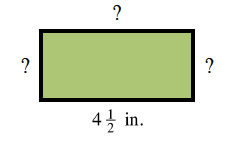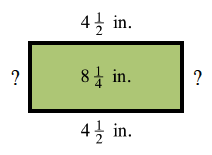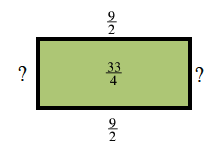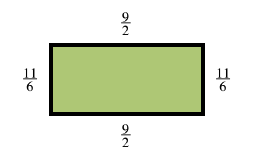### Home > CC1 > Chapter 7 > Lesson 7.1.2 > Problem7-20

7-20.

The area of the rectangle at right is $8\frac{1}{4}$ square inches. Find the perimeter. Show your work.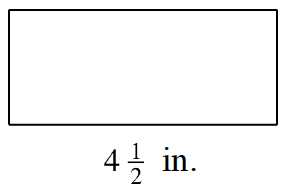1. The area is the length times the width.
2. The perimeter is the distance around the outside of the shape.

Copy the problem and label what you need to find. A rectangle with a length of 4 and one half inches. The other sides are labeled with a question mark.

Label the area and the other known side. Changed on the rectangle: 4 and one half inches, on top, instead of question mark, and 8 and one quarter square inches, in the interior of the rectangle.

Convert the fractions to improper fractions. Changed on the rectangle: 9 halves, instead of 4 and 1 half, on top and bottom, 33 fourths, instead of 8 and 1 fourth, in the interior.

Find the width.
Then add all of the sides to find the perimeter. Changed on the rectangle: 11 sixths on the right and left side, instead of question marks.